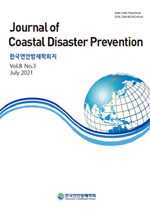상세검색
최근 검색어 전체 삭제
다국어입력
즐겨찾기0KCI등재 학술저널

# Non-Gaussian 파형경사 확률분포가 항 외곽시설 피복석 파괴 확률에 미치는 영향On the Effects of Non-Gaussian Wave-Slope Distribution on the Failure Probability of an Armour block of Rubble Mound Breakwater

• 등재여부 : KCI등재
• 2021.07
• 165 - 179 (15 pages)

This study examined the effect of non-Gaussian wave slope distribution on the failure probability of an armoring rock of rubble mound breakwater using numerical simulation. Numerical simulation was carried out using the Van der Meer equation and Level III reliability design method based on the Monte Carlo simulation. In doing so, modified Glukhovskiy wave height distribution and situ wave height data collected at Ulleungdo from January 1, 1979-December 31, 2019 were used. Tri-modal Gaissuian wave slope distribution was also used, which showed good agreements with situ wave data collected at Mangbang from April 26, 2017, to April 20, 2018. The probability coefficients of tri-modal Gaussian distribution were estimated using Matlab-based statistics and machine learning toolbox, MLE [Maximum Likelihood Estimates]. In the numerical simulation, the intrinsic limitations of Gaussian distribution were revealed, such as imposing a non-negligible probability mass even in the negative wave slope, under-shooting in longer and shorter waves, and over-shooting in mid-scale waves. In the case of failure probability of an armoring rock, Gaussian distribution was shown to give underestimated failure probability. The extent of underestimation was more considerable at Mangbang, where a tidal terrace of lower depth and gently varying slope was developed than at Ulleungdo. These differences were triggered by the presence of probability plateau formed in wave slope distribution whenever infra-gravity waves appear in random wave packet due to the resonant wave-wave interaction. Therefore, it can be easily conceivable that probability plateau has a significant effect on the armoring rock failure probability. Therefore, PIANC s recommendation that wave slope follows Gaussian distribution needs to be amended. Moreover, the reliability-based design of breakwater should be implemented based on tri-modal Gaussian wave slope distribution, which can accurately reproduce probability plateau.

1. 서론

2. 피복석 설계

3. 확률모형

4. Non-Gaussian 파형경사 분포와 Van der Meer 식에 준거한 호안 구조물 피복석 Level III 신뢰성 설계

5. 결론